Robert L. Foote
Selected Papers

• Tractrices, Bicycle Tire Tracks, Hatchet Planimeters, and a 100-year-old Conjecture, with M. Levi and S. Tabachnikov, Amer. Math. Monthly, 120 (2013) 199-216.
• Infinitesimal Isometries Along Curves and Generalized Jacobi Equations, with C.K. Han and J.W. Oh,  J Geometric Analysis, 23 (2013) 377–394.
• Area Without Integration: Make Your Own Planimeter, with Ed Sandifer, in Hands-On History: A Resource for Teaching Mathematics, MAA Notes Series #72, 2007, pp. 71-88.
• The Volume Swept Out by a Moving Planar Region, Mathematics Magazine, 79 (2006) 289-297.
• The Dynamics of Pendulums on Surfaces of Constant Curvature, with P. Coulton and G. Galperin, Mathematical Physics, Analysis and Geometry, 12 (2009), 97-107.
• Integral-Geometric Formulas for Perimeter in S2, H2, and Hilbert Planes, with R. Alexander and I.D. Berg, Rocky Mountain Journal of Mathematics, 35 (2005) 1825-1860.
• Geometry of the Prytz Planimeter, Reports on Mathematical Physics, 42 (1998) 249-271.
• Planimeters and Isoperimetric Inequalities on Constant Curvature Surfaces, in preparation.
• Dynamic, Interactive Hyperbolic Geometry with PoincaréDraw, unpublished.
Written for the conference "Exploring Undergraduate Algebra & Geometry with Technology" held at DePauw University, June 1996.
• Close Encounters with Mathematical Cranks of the Third Kind, review of Mathematical Cranks and A Budget of Trisections (renamed The Trisectors) by Underwood Dudley,
Skeptical Inquirer, Vol.18, Winter 1994, 182-186.
• Vector Bundles Over Homogeneous Spaces and Complete Locally Symmetric Spaces, with Lance D. Drager, Proc. Symp. Pure Math., 54 (1993), Part 2 ,Green and Yau, eds., 183-189.
• A Geometric Solution to the Cauchy Problem for the Homogeneous Monge-Amperè Equation, Proc. Workshops in Pure Math. Vol. 11, 1991, 31-39, Korean Acad. Council.
• Observing the Heat Equation on a Torus Along a Dense Geodesic with L. D. Drager and C. F. Martin, Sys. Sci. and Math. Sci., 4 (1991) 186-192.
• Homogeneous Complex Monge-Amperè Equations and Algebraic Embeddings of Parabolic Manifolds, Indiana Univ Math. J., 39 (1990) 1245-1273.
• Controllability of Linear Systems, Differential Geometry of Curves in Grassmannians and Generalized, Grassmannians, and Riccati Equations,
with L. D. Drager, C. F. Martin, and J. Wolper, Acta Appl. Math., 16 (1989) 281-317.
• Observing Ergodic Translations on Compact Abelian Groups with Discontinuous Functions with L.D. Drager and D. McMahon, IMA J. Math. Control & Info., 6 (1989) 441-463.
• Differential Geometry of Real Monge-Amperè Foliations, Math. Zeit., 194 (1987) 331-350.
• The Contraction Mapping Lemma and the Inverse Function Theorem in Advanced Calculus, with L. D. Drager, Amer. Math. Monthly, 93 (1986) 52-54.
• Regularity of the Distance Function, Proc. Amer. Math. Soc., 92 (1984) 153-155.
• Curvature Estimates for Monge-Amperè Foliations, Ph.D. Dissertation, University of Michigan, 1983.

Tractrices, Bicycle Tire Tracks, Hatchet Planimeters, and a 100-year-old Conjecture
with M. Levi and S. Tabachnikov
Amer. Math. Monthly, 120 (2013) 199-216.

Geometry of the tracks left by a bicycle is closely related to the so-called Prytz planimeter and with linear fractional transformations of the complex plane. We describe these relations, along with the history of the problem, and give a proof of a conjecture made by Menzin in 1906.  Reprint

• Infinitesimal Isometries Along Curves and Generalized Jacobi Equations
with C.K. Han and J.W. Oh
J Geometric Analysis, 23 (2013) 377–394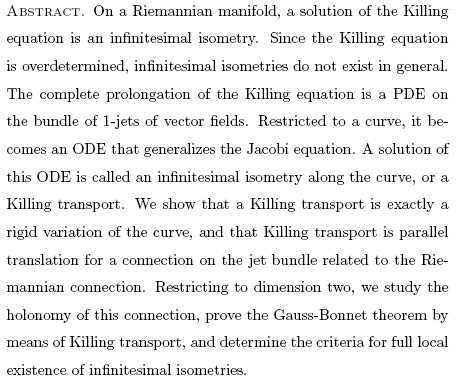arXiv Preprint

The Volume Swept Out by a Moving Planar Region
Mathematics Magazine, 79 (2006) 289-297.

A result is presented, evidently due to Courant, about the connection between the volume swept out by a moving planar region and the motion of its centroid. This generalizes both the Theorem of Pappus and Cavalieri's Principle.    Reprint

The Dynamics of Pendulums on Surfaces of Constant Curvature
with P. Coulton and G. Galperin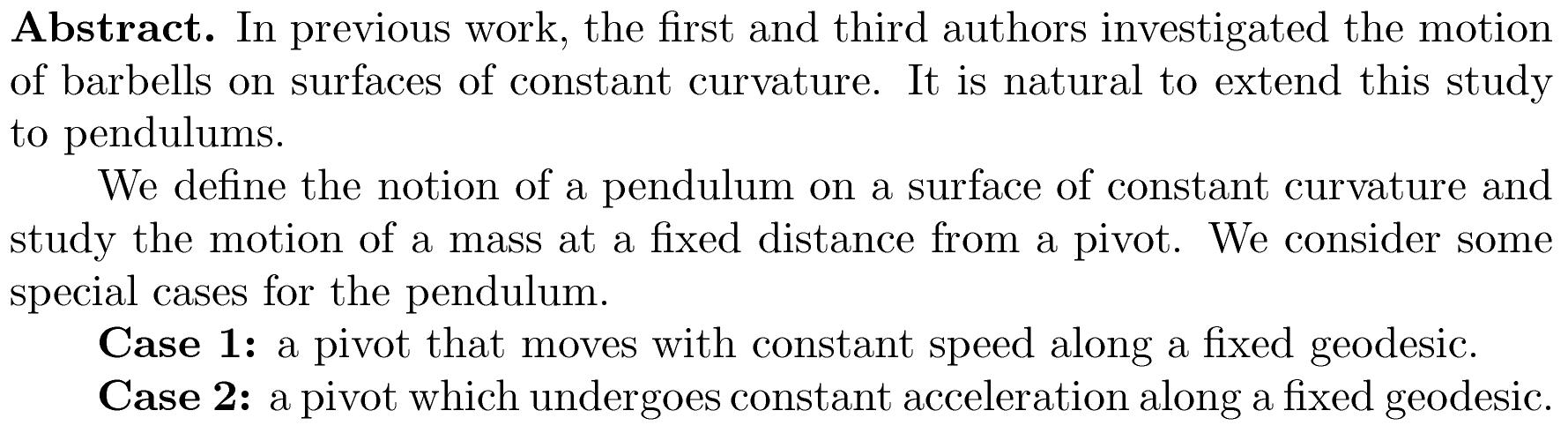Integral-Geometric Formulas for Perimeter in S2, H2, and Hilbert Planes
with Ralph Alexander and I.D. Berg
Rocky Mountain Journal of Mathematics, 35 (2005) 1825-1860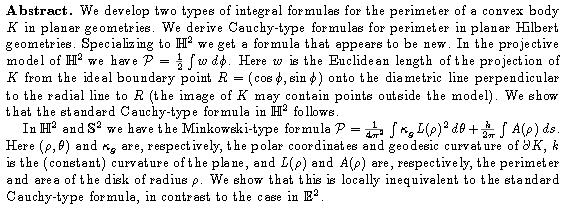Reprint

Geometry of the Prytz Planimeter
Reports on Mathematical Physics, 42 (1998) 249-271

Abstract. The Prytz planimeter is a simple example of a system governed by a non-holonomic constraint. It is unique among planimeters in that it measures something more subtle than area, combining the area, centroid and other moments of the region being measured, with weights depending on the length of the planimeter. As a tool for measuring area, it is most accurate for regions that are small relative to its length.
The configuration space of the planimeter is a non-principal circle bundle acted on by SU(1,1) ( » SL(2,R)). The motion of the planimeter is realized as parallel translation for a connection on this bundle and for a connection on a principal SU(1,1)-bundle. The holonomy group is SU(1,1). As a consequence, the planimeter is an example of a system with a phase shift on the circle that is not a simple rotation.
There is a qualitative difference in the holonomy when tracing large regions as opposed to small ones. Generic elements of SU(1,1) act on S1 with two fixed points or with no fixed points. When tracing small regions, the holonomy acts without fixed points. Menzin's conjecture states (roughly) that if a planimeter of length l traces the boundary of a region with area A > pl2, then it exhibits an asymptotic behavior and the holonomy acts with two fixed points, one attracting and one repelling. This is obvious if the region is a disk, and intuitively plausible if the region is convex and A >> pl2. A proof of this conjecture is given for a special case, and the conjecture is shown to imply the isoperimetric inequality.
Presented at the Pacific Insitute for the Mathematical Sciences Workshop on Non-holonomic Constraints in Dynamics, Calgary, August 1997, and at the Lehigh University Geometry & Topology Conference, June 1998.

Planimeters and Isoperimetric Inequalities on Constant Curvature Surfaces
In preparation.  Preliminary version available.

Abstract.  A planimeter is a mechanical instrument used to determine the area of a region in the plane.  As the boundary of the region is traced, a wheel attached to the instrument partially rolls and partially slides, recording a component of its motion on the plane. The area of the region is a simple function of the net roll of the wheel.  We show how the analogue of this instrument works on the sphere and the hyperbolic plane, and then use the results to give a simple proof of one of the Bonnesen isoperimetric inequalities for these surfaces.  The rolling of the wheel can be interpreted as parallel translation for a connection in a certain bundle over the surface. The isoperimetric inequality can then be viewed as a statement about the holonomy of this connection.

Presented at Lehigh Geometry/Topology Conference, June 1997

Dynamic, Interactive Hyperbolic Geometry with PoincaréDraw

An account of the motivation for and development of the computer program PoincaréDraw. Unpublished. Written for the conference "Exploring Undergraduate Algebra & Geometry with Technology" held at DePauw University, June 1996.

Complete text.

Close Encounters with Mathematical Cranks of the Third Kind
review of
Mathematical Cranks and A Budget of Trisections (renamed The Trisectors) by Underwood Dudley
Skeptical Inquirer, Vol.18, Winter 1994, 182-186.

For better or for worse, pseudo-mathematics does not share the popularity among cranks that other subjects seem to have. It just doesn't have the same appeal as parapsychology, astrology, or UFOs.  Nevertheless, mathematical cranks do exist, as mathematics departments at many major universities are well aware. When I was a graduate student at the University of Michigan, it was not uncommon for the departmental mailboxes to be stuffed periodically with the local crank's most recent proof of the rationality of p or the square root of 2.
While most crank mathematics winds up in the wastebasket (frequently after serving as departmental entertainment), occasionally a well-meaning mathematician makes the mistake of responding to a crank, pointing out his errors, hoping to set him straight. Thus may begin a series of communications between crank and mathematician (mostly from crank to mathematician), usually ending when the mathematician is forced to be rude, or the crank becomes angry and goes in search of a more "tolerant" mathematician.  The moral of the story is, don't get involved with a crank.
While frequently giving this advice to others, Underwood Dudley, professor of mathematics at DePauw University in Greencastle, Indiana, has made it his professional hobby to collect the works of modern mathematical cranks, to correspond with them, and even to meet them. In these two books, he shares his fascinations and frustrations with people bent on pseudo-mathematics.
What types of things do mathematical cranks work on? Most of Dudley's examples can be placed into two broad categories: 1) real mathematics that has been twisted, misunderstood, or has had its significance horribly exaggerated, and 2) impenetrable nonsense.
Excerpt from the review.

Vector Bundles Over Homogeneous Spaces and Complete Locally Symmetric Spaces
with Lance D. Drager
Proceedings of Symposia in Pure Mathematics, 54 (1993), Part 2, Green and Yau, eds., 183--189.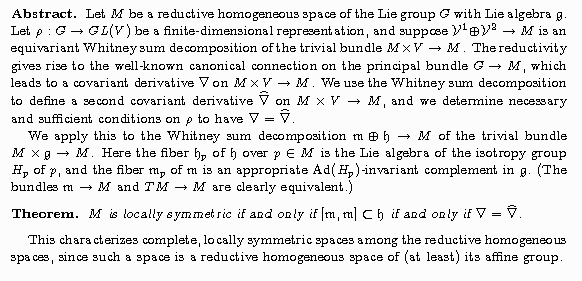A Geometric Solution to the Cauchy Problem for the Homogeneous Monge-Amperè Equation
Proc. Workshops in Pure Math. Vol. 11, 1991, 31--39, Korean Acad. Council.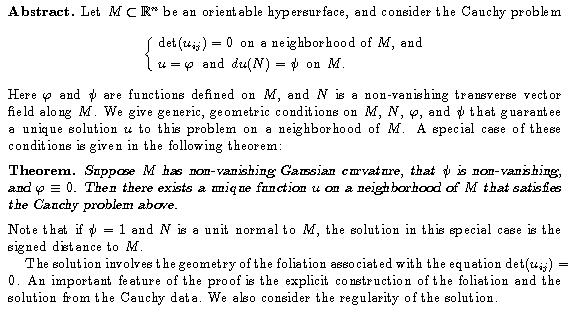Reprint

Observing the Heat Equation on a Torus Along a Dense Geodesic
with L. D. Drager and C. F. Martin
Sys. Sci. and Math. Sci., 4 (1991) 186--192

Abstract.  Several authors have considered observability problems for the heat equation and related partial differential equations. A basic problem is to determine what kinds of sampling provide sufficient information to uniquely determine the initial heat distribution. We address the case where the temperature is measured while traveling along a curve.
We consider the special case where the space is a flat torus (of arbitrary dimension) and the curve is a geodesic. It is shown that, in this case, the observed temperature is sufficient information to uniquely determine the initial heat distribution if and only if the geodesic is dense in the torus.
In the case of a torus, Fourier analysis techniques can be used to write down the solution of the heat equation. This allows us to derive an explicit representation of the observed temperature in terms of the initial distribution. We use this representation and some ideas from the theory of almost periodic functions to show that the Fourier coefficients of the initial distribution can be recovered from the observation.

Homogeneous Complex Monge-Amperè Equations and Algebraic Embeddings of Parabolic Manifolds
Indiana Univ Math. J., 39 (1990) 1245-1273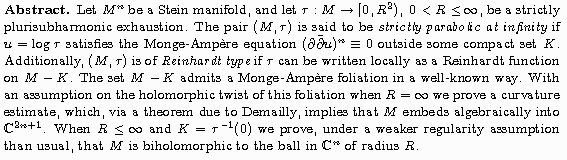Reprint

Controllability of Linear Systems, Differential Geometry of Curves in
Grassmannians and Generalized Grassmannians, and Riccati Equations
with Lance D. Drager, Clyde F. Martin, and James Wolper
Acta Appl. Math., 16 (1989) 281-317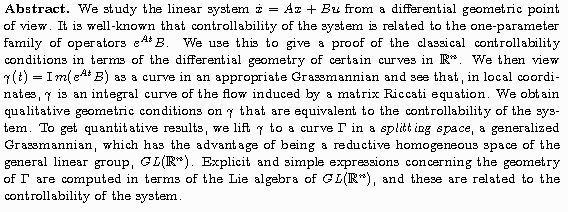Observing Ergodic Translations on Compact Abelian Groups with Discontinuous Functions
with L.D. Drager and D. McMahon
IMA J. Math. Control & Info., 6 (1989) 441--463

Abstract. Several authors have considered the problem of global observability for minimal dynamical systems, and particularly the example of ergodic (equivalently, minimal) translations on compact abelian groups. The global observability problem for ergodic translations is considered here in the case where the output function may be discontinuous. The result for continuous output functions, essentially due to McMahon, is described. This result shows that a continuous output function observes all ergodic translations if and only if it has no nontrivial symmetries. This result is extended here in two directions.
First, functions continuous except on a meagre set and symmetries  modulo meagre sets are considered. A result analogous to the continuous case is obtained that includes and generalizes the continuous case and results of Balogh, Bennett, and Martin on observing ergodic translations with the characteristic functions of certain subsets of the group. Functions continuous except on a set of measure zero are also considered.
Secondly, ergodic theory and harmonic analysis techniques are employed to consider the case of integrable output functions and symmetries modulo sets of measure zero. It is shown that an integrable function observes ergodic translations modulo sets of measure zero if and only if it has no nontrivial symmetries modulo sets of measure zero.
The group of symmetries of the output function modulo sets of measure zero can be determined from its Fourier series. The results here show that classical observability can be determined from the Fourier series when the function is continuous almost everywhere, but only observability modulo sets of measure zero can be determined when the output function is merely integrable.

Differential Geometry of Real Monge-Amperè Foliations
Mathematische Zeitschrift, 194 (1987) 331-350

Abstract. The foliations studied in this paper are those that arise from the Monge-Amperè condition requiring the Hessian of a function defined on an affine manifold to have rank that is one less than maximal.  The real, homogeneous Monge-Amperè equation is  det H(u) = 0, where u is a real-valued function on an open subset of Rn.  Solutions that satisfy  rank H(u) = n-1  induce a foliation of the domain of u by lines. The standard solution is u(x) = ||x||, which satisfies  rank H(u) = n-1.  The standard solution is characterized in three ways using the geometric "twist" of the foliation, the covariant derivatives of H(u), and strictly convex exhaustions of the domain of u.  The Cauchy problem for  det H(u) = 0  is treated.

The Contraction Mapping Lemma and the Inverse Function Theorem in Advanced Calculus
with Lance D. Drager
Amer. Math. Monthly, 93 (1986) 52-54

Abstract. The most elegant and general proof of the inverse function theorem uses the contraction mapping lemma. In an advanced calculus course, time does not generally permit a proof of the contraction mapping lemma as it is usually formulated. We give a simple proof of the contraction mapping lemma in Rn based on the max/min principle, familiar to calculus students -- a continuous, real-valued function defined on a non-empty, closed, bounded subset of Rn attains its maximum and minimum on that set -- thus allowing the general proof of the inverse function theorem usually left for more advanced courses.

Reprint

Regularity of the Distance Function
Proc. Amer. Math. Soc., 92 (1984) 153-155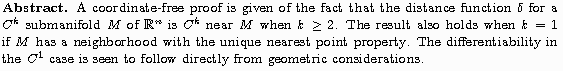Reprint

Curvature Estimates for Monge-Amperè Foliations
Ph.D. Dissertation, University of Michigan, 1983

Abstract. The foliations studied in this paper are those that arise from the Monge-Amperè condition that requires the Hessian of a real-valued function to have rank that is one less than maximal.
In the first chapter, the geometry of the foliations of Rn is studied. The main theorems are the two characterizations of the standard foliation.
In the second chapter, the foliations of complex manifolds are studied. The locally Reinhardt foliations are those to which the geometry and methods of the first chapter can be applied directly. Hörmander's L2- technique is used to relate the geometry and the analysis of a Stein manifold that admits such a foliation outside a compact set. The main result is the embedding theorem of the last section.

Last updated 14 May 2014
Robert Foote